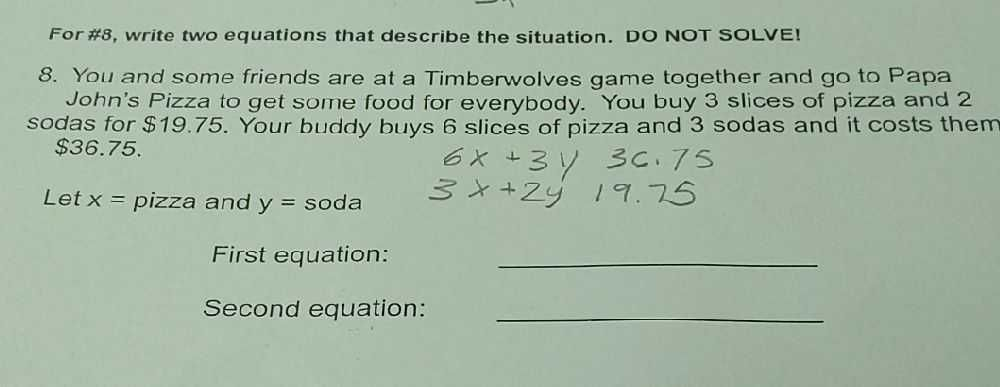### ¿Todavía tienes preguntas de matemáticas?

Pregunte a nuestros tutores expertos
Algebra
Pregunta8. You and some friends are at a Timberwolves game together and go to Papa John's Pizza to get some food for everybody. You buy $$3$$ slices of pizza and $$2$$ sodas for $$\ 19.75$$ . Your buddy buys $$6$$ slices of pizza and $$3$$ sodas and it costs them $$\ 36.75 .$$

Let $$x =$$ pizza and $$y =$$ soda First equation: Second equation: